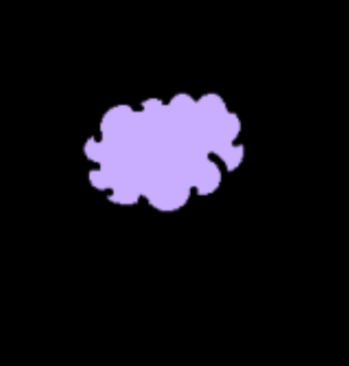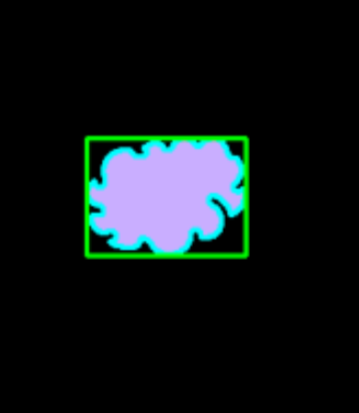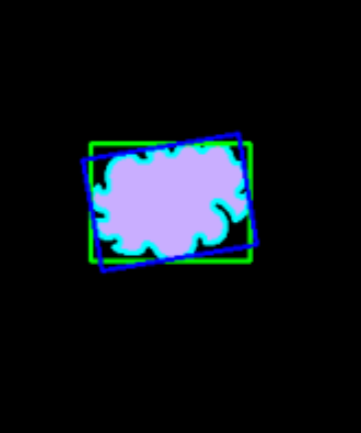GeeksforGeeks App
Open AppBrowser
Continue

# Finding minimum enclosing rectangle in OpenCV Python

In this article, we are going to see how to draw the minimum enclosing rectangle covering the object using OpenCV Python. For that, we will be using the concepts of Contours

In this step, we will import the OpenCV and NumPy library and then read the image with its help.

## Python3

 `import` `cv2``import` `numpy as np`` ` `# To read image``img ``=` `cv2.imread(``"cloud.png"``, cv2.IMREAD_COLOR)`

Output:### Convert image to grayscale

To get a clear image we need to convert it into grayscale.

## Python3

 `gray ``=` `cv2.cvtColor(img, cv2.COLOR_BGR2GRAY)``ret, thresh ``=` `cv2.threshold(gray, ``127``, ``255``, ``0``)`

### Find the contours in the image

Contours can be extracted by using cv2.findContours() function. We can select a contour cnt or loop over the all contour

## Python3

 `# finding the contours``contours, _ ``=` `cv2.findContours(thresh, cv2.RETR_TREE,``                               ``cv2.CHAIN_APPROX_SIMPLE)`` ` `# take the first contour``cnt ``=` `contours[``0``]`

### Drawing and Displaying Rectangle Boundaries

In this step, we will see how the rectangle will cover the cloud in the image.

## Python3

 `# computing the bounding rectangle of the contour``x, y, w, h ``=` `cv2.boundingRect(cnt)`` ` `# draw contour``img ``=` `cv2.drawContours(img, [cnt], ``0``, (``0``, ``255``, ``255``), ``2``)`` ` `# draw the bounding rectangle``img ``=` `cv2.rectangle(img, (x, y), (x``+``w, y``+``h), (``0``, ``255``, ``0``), ``2``)`` ` `# display the image with bounding rectangle drawn on it``cv2.imshow(``"Bounding Rectangle"``, img)``cv2.waitKey(``0``)``cv2.destroyAllWindows()`

Output:### Minimum Enclosing Rectangle

Now, let’s talk about the minimum enclosing rectangle. Is it the minimum size? To check this, let’s use the minAreaRect() function and OpenCV.

## Python3

 `rect ``=` `cv2.minAreaRect(cnt)``box ``=` `cv2.boxPoints(rect)``box ``=` `np.int0(box)``img ``=` `cv2.drawContours(img, [box], ``0``, (``0``, ``0``, ``255``), ``2``)`` ` `# display the image with bounding rectangle drawn on it``cv2.imshow(``"Bounding Rectangle"``, img)``cv2.waitKey(``0``)``cv2.destroyAllWindows()`

Output:My Personal Notes arrow_drop_up